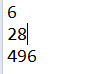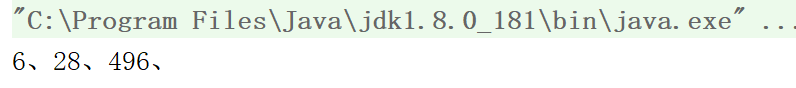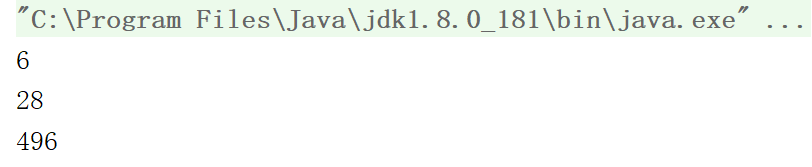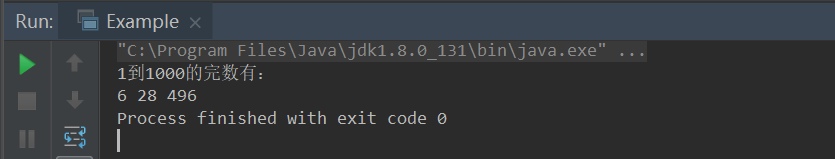• 编程找出 1000 以内的所有完数。 for(int num=1;num<=1000;num++){ int total=0; for(int yinZi=1;yinZi<num;yinZi++){ if(num%yinZi==0){ //计算因子和过程 total+=yinZi; } } if...

一个数如果恰好等于它的因子之和，这个数就称为“完数”。例如 6 = 1＋2＋3。编程找出 1000 以内的所有完数。

for(int num=1;num<=1000;num++){
int total=0;
for(int yinZi=1;yinZi<num;yinZi++){
if(num%yinZi==0){ //计算因子和过程
total+=yinZi;
}
}
if(total==num){ //判断因子和和自身是否相等
System.out.println(num);
}
}

6
28
496

展开全文java 算法
• 一个数如果恰好等于它的因子之和，这个数就称为 "完数 "。例如6=1＋2＋3.编程找出1000以内的所有完数Java 完数
• JAVA求1000以内的完数

万次阅读 多人点赞 2018-05-12 10:54:45
编写应用程序求1000之内的所有完数。 public class Hello { public static void main(String[] args){ int i,j; int sum=0; //记录因子之和 for(i=1;i&lt;=1000;i++) { for(j...

一个数如果恰好等于它的因子之和，这个数就成为完数。编写应用程序求1000之内的所有完数。

public class Hello {
public static void main(String[] args){
int i,j;
int sum=0;        //记录因子之和
for(i=1;i<=1000;i++) {
for(j=1;j<i;j++) {
if(i%j==0)
sum=sum+j;
}
if(sum==i)    //如果因子之和等于它本身，就输出
System.out.println(i);
sum=0;
}
}
}

结果：展开全文• java编程找出1000以内的完数

千次阅读 2019-03-19 21:11:45
一个数如果恰好等于它的因子之和，这个数就称为 ...编程 找出1000以内的所有完数。 直接上代码： public class Test{ public static boolean sum(int num){ int temp = 0; for(int i = 1;i < num;i++){ if(n...

一个数如果恰好等于它的因子之和，这个数就称为 "完数 "。例如6=1＋2＋3。编程 找出1000以内的所有完数。
直接上代码：

public class Test{
public static boolean sum(int num){
int temp = 0;
for(int i = 1;i < num;i++){
if(num%i==0){
temp += i;
}
}
if(temp==num){
return true;
}
return false;
}
public static void main(String[] args) {
Test test = new Test();
for(int i = 1;i < 1000;i++){
if(test.sum(i)){
System.out.print(i + "、");
}
}
}
}

运行结果：另一种解法：

public class Test{
public static void main(String[] args) {
for(int i = 2;i <=1000;i++){
int temp = 0;
for(int j = 1;j < i;j++){
if(i%j == 0){
temp += j;
}
}
if(temp == i){
System.out.println(temp);
}
}
}
}

运行结果：展开全文• 题目： 一个数如果恰好等于它的...编程 找出1000以内的所有完数。 代码如下： package mytest; public class Example { public static void main(String[] args) { System.out.println("1到1000完数有："); ...

题目： 一个数如果恰好等于它的因子之和，这个数就称为 "完数 "。例如6=1＋2＋3.编程 找出1000以内的所有完数。

代码如下

package mytest;

public class Example {
public static void main(String[] args) {
System.out.println("1到1000的完数有：");
for (int i=1; i < 1000; i++){
int t = 0;
for (int j=1; j <= i/2; j++){
if(i % j == 0){
t = t + j;
}
}
if(t == i){
System.out.print(i+" ");
}
}
}
}

执行结果如下图：展开全文Java经典编程案例
• java求100以内的完数

千次阅读 2020-08-03 17:50:43
java 100以内完数 什么是完数： 一个数正好等于它的因子之和，就叫做完数 因子就是除去这个数本身的其它约数 代码： public class Main { public static void main(String[] args) { for (int i = 1; i < ...java 编程语言
• 求1000之内的所有完数 完数：一个数刚好等于它的因子之和。 思路分析：对每个数从1的该数之间寻找因子(类似于素数的找因子)，将该数的所有因数进行相加所得的和与该数进行比较。若相等，则其为完数。 代码入下： ...java
• 实现思路 1、建立外层For循环，获取1~1000完数的循环,定义int i=1，通过i++,实现i为1~1000的所有整数。 2、进行For循环嵌套，定义整型int i的因子int j=1，j++。在内层For循环中，通过if条件语句(i%j)判断1~i中int...java 开发语言 eclipse
• 编程：找出1000以内的所有完数。（因子：除去这个数本身的其他约数。） 直接上代码： public class PerfectNumber{ public static void main(String[] args) { for (int i=1; i<=1000; i++){ int factor = 0;...java jdk
• 展开全部#include void main(){int i,j;printf("1000之内的所有完数：\n");for(i=6;i
• 编程找出1000以内的所有完数。 我的思路： 1、for循环，给 i 赋值1~1000 2、找出能整除 i 的数并相加 3、相加之后的数sum等于 i 的话，就输出 i 代码如下： package com.thz.hnstc.test01; /* * @author NanTang * *...java
• 利用Java如何找出1000以内的完全数发布时间：2020-11-10 16:30:52来源：亿速云阅读：101作者：mdxy-dxy本篇文章为大家展示了利用Java如何找出1000以内的完全数，内容简明扼要并且容易理解，绝对能使你眼前一亮，通过...
• 编程找出1000以内的所有完数

千次阅读 2019-01-21 22:27:38
编程找出1000以内的所有完数。 2.一共两种方法 1） //int数组+两层for循环. 空间复杂度与时间复杂度都是最高 private static void method1(){ //数组记录完数 int[] p = new int; ...Java基础
• 编程找出1000以内的所有完数，并按下面格式输出： 6 its factors are 1,2,3 public class test { public static void main(String[] args) { for (int i = 1; i <= 1000; i++) { int x = i;java 程序人生 经验分享
• public class Homework01{ public static void main(String[] args) { int i, j, k for(i=1; i<1000; i++){ if(i%j==0){ ...
• public class SumNum {public static void main(String[] args) {System.out.println("1000以内完数如下：");fun();}//求完数完数的定义：一个数恰好等于它的所有因子之和public static void fun(){for(int i=1;...
• /*项目:用Java编程实现1000以内的所有完全数 (如果一个自然数 N 恰好等于它所有不同的真因子（即 N 的约数以及 1，但不包括 N）之和 S， 则称该数为“完美数”。例如 6＝1＋2＋3，28＝1＋2＋4＋7＋14,所以6和28都是...
• 版权声明：本文为博主原创文章，未经博主允许不得转载。 /* (程序头部注释开始) ...* 文件名称： 《一些简单的java编程题(1) ————10000以内完数》 * 作 者： 那就去巴黎 * 完成日期： 2018 年 01 月 2java 完数 编程
• 编程找出1000以内的所有完数。 程序设计： 根据定义，要找出1000之内的完数，需要将循环控制在1到1000，然后再判断这个数是不是完数（先出这个数的所有因子，将这些因子相加得到的和与该数进行比较，如果相等...完数
• 编程找出1000以内的所有完数。(学习视频分享：java教学视频)思路：for循环，给 i 赋值1~1000找出能整除 i 的数并相加相加之后的数sum等于 i 的话，就输出 i实现代码：package com.thz.hnstc.test01;/** @author ...
• 一个数如果恰好等于它的因子之和，这个数就称为 "完数 "。例如 6=1＋2＋3.编程 找出 1000 以内的所有完数java 算法
• 编程输出1000以内所有的完数，并输出其所有因子。所谓完数，即一个数的所有因子（除其自身）之和恰好等于其自身。如6就是一个完数，6=1+2+3。 **输出格式要求："\n%5d\n", "%5d" 程序运行示例如下： 6 1 2 3 28 ...
• 1000以内的所有完数

千次阅读 2018-06-09 22:03:10
题目：一个数如果恰好等于它的因子之和，这个...编程找出1000以内的所有完数。public class Prog9{ public static void main(String[] args){ int n = 10000; compNumber(n); } //求完数 private static void ...
• 一个数如果恰好等于它的因子之和，这个数就被称作为“完数”。例如6=1+2+3 package p3; public class perfectnumber { public static void main(String[] args)... System.out.println("1到1000完数有："); ...java...

编程求1000以内的完数javajava 订阅